12 4x)x,is an another form of representation of fuzzy set.A, (x) /x, is an another form of representation of fuzzy setRepresent the following fuzzy sets by this form.

Question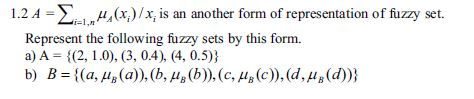help_outlineImage Transcriptionclose12 4x)x,is an another form of representation of fuzzy set. A, (x) /x, is an another form of representation of fuzzy set Represent the following fuzzy sets by this form. fullscreen
Step 1

A fuzzy set is a pair X, m(A)) where X is a Universal set and mA) is a membership function or degree of membership function. Now let  us solve the given  problem one by one:

1. a) A={(2,1.0),(3,0.4),(4,0.5)}

From the given information, you can   conclude that

we have a universal set X={2,3,4} and a degree of membership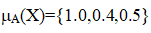Given information can be represented as: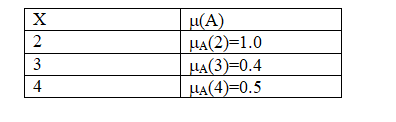Step 2

For the sake of convenience, usually a fuzzy set is represented as: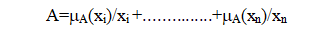or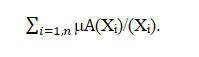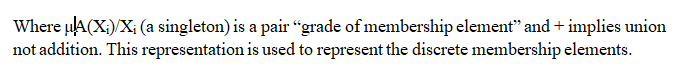Step 3

Since the elements are discrete, we can represent this as: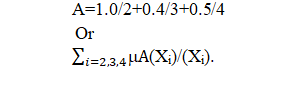...

Want to see the full answer?

See Solution

Want to see this answer and more?

Our solutions are written by experts, many with advanced degrees, and available 24/7

See Solution
Tagged in

Computer Science